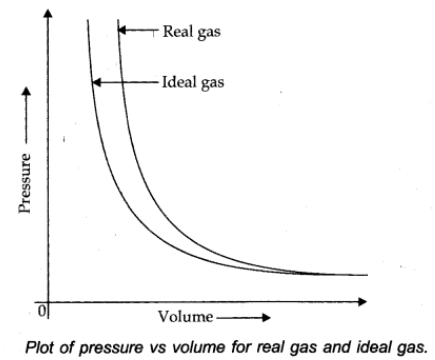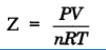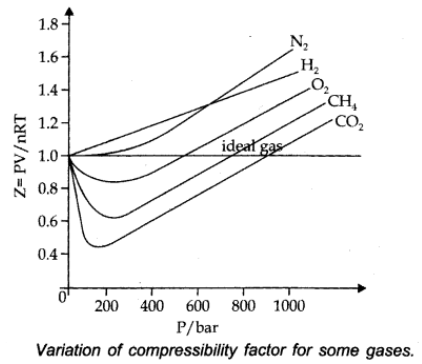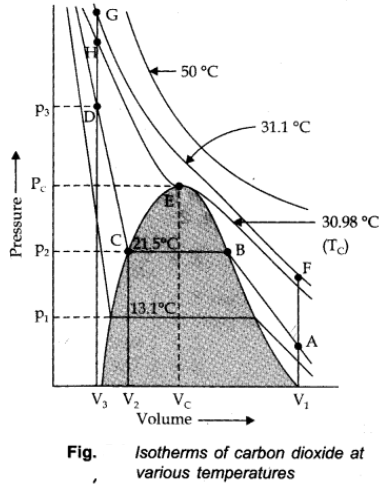# Real Gas

A gas which does not follow ideal gas behavior under all conditions of temperature and pressure is called real gas.

Deviation with respect to pressure can be studies by plotting pressure Vs volume curve at a given temperature (Boyle’s law)### Compressibility factor (Z)

Deviation from ideal behavior can be measured in terms of compressibility factor, Z.For ideal gas Z = 1

For real gas Z ≠ 1

when Z > 1 Real gases show positive deviation from ideality

Z< 1 The gas show more compressibility and deviation are regarded as negative deviation.## Van der Waals Equationwhere V is a constant for a molecular attraction while V is a constant for molecular volume.

1. There is no force of attraction between the molecules of a gas.
2. The volume occupied by the gas molecule is negligible in comparison to the total volume of the gas.

Above two assumptions of the kinetic theory of gas was found to be wrong at very high pressure and low temperature.

# Liquefaction of Gases

Liquefaction of gases can be achieved either by lowering the temperature or increasing the pressure of the gas simultaneously.

Thomas Andrews plotted isotherms of CO2 at various temperatures shown in figure.### Critical Temperature (TC):

It is defined as that temperature above which a gas cannot be liquified however high pressure may be applied on the gas.

TC = 8a/27bR

### Critical Pressure (PC):

It is the pressure required to liquify the gas at the critical temperature.

Pc = a/27b2

### Critical volume (VC):

The volume occupied by a mole of the gas at the critical temperature and the critical pressure is called the critical volume (VC).

For example: For CO2 to Liquify

TC = 30.98 oC

PC = 73,9 atm

VC = 95-6 cm3/mole

All the three are collectively called critical constants